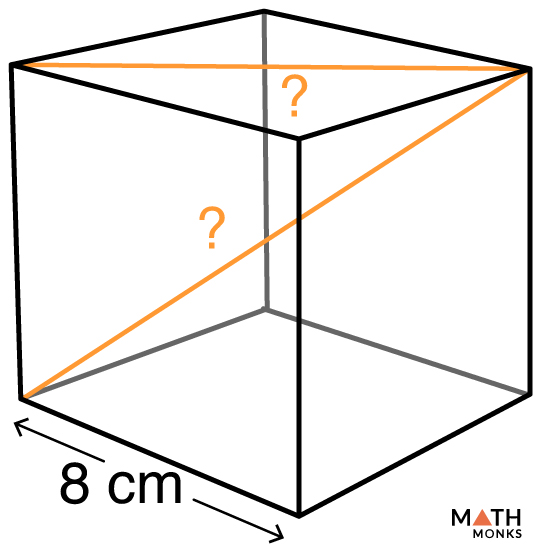# Diagonal of a Cube

The diagonal of a cube is the straight line connecting any of its two non-adjacent vertices. It is also called the body or space diagonal since it passes through the center of a cube. There are 4 body diagonals in a cube. Since the diagonal is a linear quantity, it is expressed in linear parameters such as mm, cm, m, in, or ft.

## Formula

The formula is:

### Derivation

Let us have a cube with an edge of ‘a’ units.

∴ AB = BD = DC = a, as shown in the figure below.

The face diagonal is the hypotenuse of the right triangle △BDC.

∴  applying Pythagoras theorem, we get,

(BC)2 = (BD)2 + (DC)2

(BC)2 = a2 + a2, since BD = DC = a

(BC)2 = 2a2  … (1)

∴ BC = √2a (here BC is the face diagonal)

So, the face diagonal of a cube = √2a

Also, in △ABC, the body diagonal AC is the hypotenuse.

∴  applying Pythagoras theorem, we get,

(AC)2 = (AB)2 + (BC)2

Let AC = d

∴ d2 = (AB)2 + (BC)2

d2 = a2 + 2a2 , [replacing (BC)2 = 2a2 from (1)]

d2 = 3a2

d = √3a

Hence, we get the formula for the diagonal of a cube as d = √3a

We also get the formula:

Face diagonal = √2a, where a = edge

The face diagonal connects two non-adjacent vertices on a face. It is not the main diagonal of a cube. There are 12 face diagonals in a cube.

## Solved Examples

Find the length of a diagonal of a cube whose edge is 6 cm.

Solution:

As we know,
The diagonal of a cube means its body diagonal,
Body Diagonal (d) = ${\sqrt{3}a}$, here a = 6 cm
∴d = √3 × 6
= 10.39 cmCalculate the diagonals of a cube as given in the figure alongside.

Solution:

As we know,
Body Diagonal (d) = ${\sqrt{3}a}$, here a = 8 cm
∴ d = √3 × 8
= 13.86 cm
Face Diagonal = ${\sqrt{2}a}$, here a = 8 cm
∴ d = √2 × 8
= 11.31 cm

Calculate the diagonal of a 3×3 Rubik’s cube.

Solution:

As we know,
Body Diagonal (d) = ${\sqrt{3}a}$, here a = 3 units
∴ d = √3 × 3
= 5.2 cm